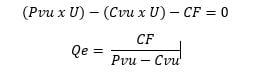# Balance Point

The Balance Point is established through a calculation that serves to define the moment in which a company’s income covers its fixed and variable expenses, that is, when you manage to sell the same as you spend, you neither win nor lose, you have reached the breakeven.

Thus, calculating the Balance Point point is essential for companies to evaluate their profitability, since in this way it is possible to know how much they need to sell to generate profit. Knowing this value, even before starting a new project, allows you to know how financially interesting your business idea is. Doing the calculation also helps you know how much time, approximately, it will take for your business to start making profits . That is, it is a fundamental stage for any business plan.

## Elements of the Balance Point

To determine a business balance Point, it is important to know when you start to really make money, since it is there when income has the ability to cover variable expenses and fixed expenses.

Knowing how much to sell is not complicated, you just have to take into account 5 key elements and the detail of the formula to calculate the balance point. The first thing you should know are your expenses, both fixed and variable, in order to know your total costs. We explain you in more detail below.

In order to calculate the equilibrium point, it is important to know its fundamental elements , with which a result can be obtained both in monetary value and in units.

### 1. Unit sales price

This element is part of the variable costs. It refers directly to the sale price of the final units.

### 2. Fixed costs

Also known as fixed expenses , they are those that do not have changes month after month, on the contrary, they have the same value every month. In this sense, they are completely independent of the billing value.

Among the fixed costs, several examples can be mentioned, one of them the rental of real estate since it is independent of the volume of sales, its value is always the same. Other examples are monthly rent, electricity costs, maintenance, or depreciation.

### 3. Variable costs

Also known as variable expenses are those that, as their name indicates, vary according to the billing value, this means that they have a direct relationship with the volume of sales. If sales increase, variable costs will do the same.

Examples of variable costs include employee commissions , rights and royalties, ISR, raw material for production, labor, etc.

### 4. Total costs

The total costs are the result of the sum of the variable costs and the fixed costs. Knowing well what your total costs are, you can easily deduct a balance Point, since it occurs when income is equal to total costs.

### 5. Unit variable cost

To calculate the value of the unit variable cost , a division of all variable costs (mentioned above) must be made by the number of units sold or services provided during a given period of time.

The formula you should use is the following:

## How does the Balance point work?

Using the formula of:We can easily know our equilibrium point. Suppose you sell shoes, if your company has a fixed cost of 6000, you sell each pair of shoes at 50 (Unit sale price) and the production of each one comes out at 20 (Unit sale cost).

You will need to sell 200 shoes to cover the total costs. That is, from 201, you will be making profits.

## What is the importance of the balance Point?

Being able to determine the importance of the balance Point is key in any type of business, since it allows you to know what the level of sales is necessary so that you can cover the total costs.

In other words, you must reach a certain level of income to be able to cover both fixed and variable expenses.

The balance Point is one of the most relevant strategic tools when determining the level of solvency that a business has, or if it has sufficient profitability.

Check Also
Close